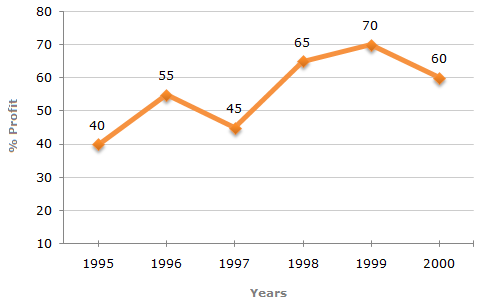# Data Interpretation - Line Charts

Exercise : Line Charts - Line Chart 6
Directions to Solve

The following line graph gives the annual percent profit earned by a Company during the period 1995 - 2000.

Percent Profit Earned by a Company Over the Years.

 %Profit = Income - Expenditure x 100 Expenditure1.
If the expenditures in 1996 and 1999 are equal, then the approximate ratio of the income in 1996 and 1999 respectively is?
1:1
2:3
13:14
9:10
Explanation:

Let the expenditure in 1996 = x.

Also, let the incomes in 1996 and 1999 be I1 and I2 respectively.

Then, for the year 1996, we have:

 55 = I1 - x x 10055 = I1 - 1I1 = 155x ... (i) x 100 x 100

 70 = I2 - x x 10070 = I2 - 1I2 = 170x ... (ii) x 100 x 100

From (i) and (ii), we get:

I1 =155x100
= 1550.919 : 10.
I2170x100
170 1

2.
If the income in 1998 was Rs. 264 crores, what was the expenditure in 1998?
Rs. 104 crores
Rs. 145 crores
Rs. 160 crores
Rs. 185 crores
Explanation:

Let the expenditure is 1998 be Rs. x crores.

 Then, 65 = 264 - x x 100 x65 = 264 - 1 100 xx = 264 x 100 = 160. 165Expenditure in 1998 = Rs. 160 crores.

3.
In which year is the expenditure minimum?
2000
1997
1996
Cannot be determined
Explanation:
The line-graph gives the comparison of percent profit for different years bu the comparison of the expenditures is not possible without more data.

Therefore, the year with minimum expenditure cannot be determined.

4.
If the profit in 1999 was Rs. 4 crores, what was the profit in 2000?
Rs. 4.2 crores
Rs. 6.6 crores
Rs. 6.8 crores
Cannot be determined
Explanation:

From the line-graph we obtain information about the percentage profit only. To find the profit in 2000 we must have the data for the income or expenditure in 2000.

Therefore, the profit for 2000 cannot be determined.

5.
What is the average profit earned for the given years?
50(2/3)
55(5/6)
60(1/6)
335